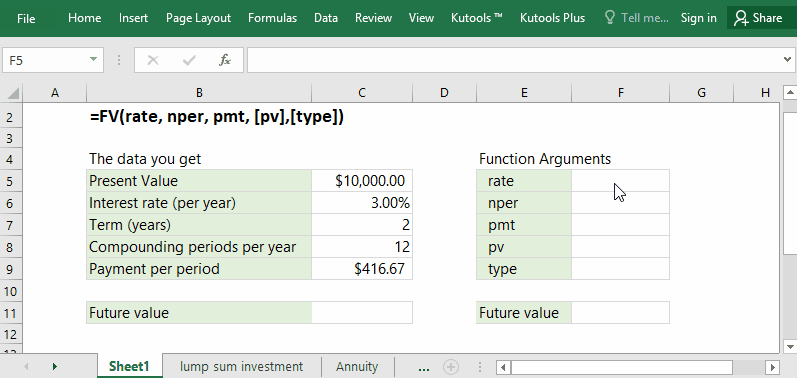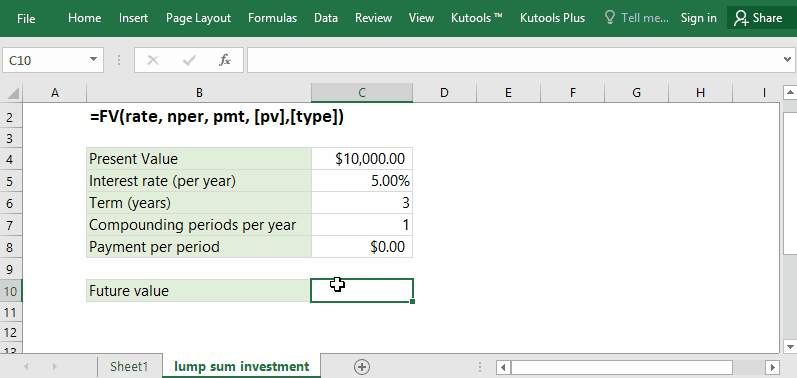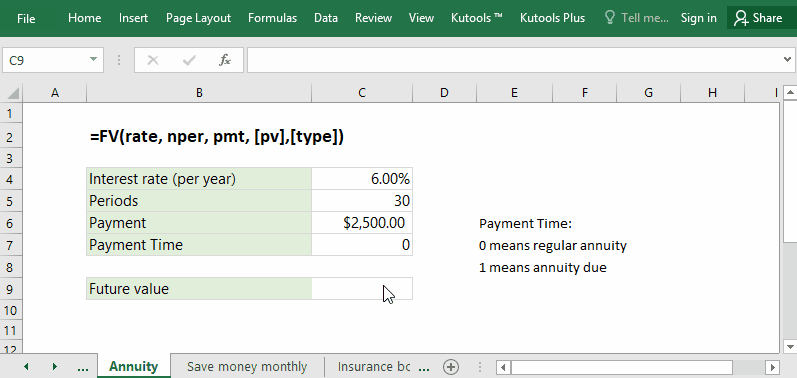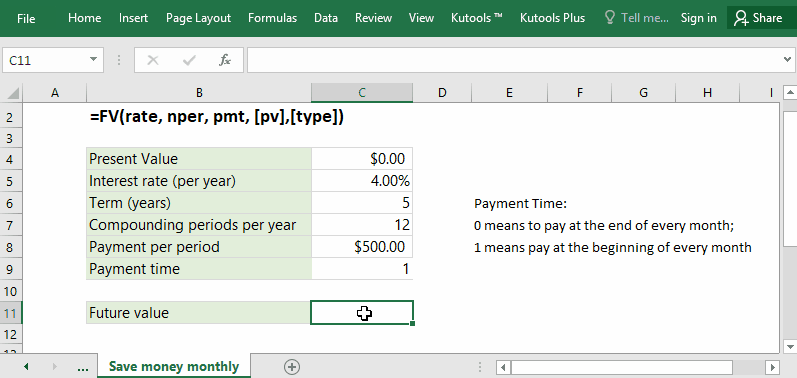Note: The other languages of the website are Google-translated. Back to English

## Excel FV Function

The FV function is a financial function in Excel, and it will figure out the future value of an investment, installment, or scheduled payments based on periodic, constant payments with fixed interest rate. And this FV function can also calculate the future values for a lump sum payment in Excel.#### Function syntax and arguments

FV(rate, nper, pmt, [pv],[type])

(1) Rate: Required. The interest rate per period. It’s constant in the whole life of the investment.

Let’s say you get the annual interest rate of 6%, you can change it as follows:

• For semi-annual payments, you need to convert it to semi-annual interest rate: 3% (=6%/2);
• For quarterly payments, you need to convert it to quarterly interest rate: 1.5% (=6%/4);
• For monthly payments, you need to convert it to monthly interest rate: 0.5% (=6%/12).

(2) Nper: Required. The total number of payment periods. Supposing you need to pay for 3 years, you can change it as follows:

• For quarterly payments, the total number of payment periods is 12 (=3*4);
• For monthly payments, the total number of payment periods is 36 (=3*12).

(3) Pmt: Required. The constant payment in each period. It’s fixed in the whole life of investment.

(4) Pv: Optional. The present value of your investment, or the lump sum payment at present. If omitted, the FV function will calculate it as 0.

(5) Type: Optional. A value tells the time of payment. There are two types:

• 0 or omitted: payment at the end of every period;
• 1: payment at the beginning of every period.

#### Return Value

Numerical value.
The FV function will return the future value of an investment based on periodic, constant payments and a fixed interest rate.

#### Usage notes

(1) Interest rate: Make sure the unit of interest rate is matched with payment periods.

(2) Constant payment in each period: if for cash out, it should be negative; while for cash received, it should be positive.

#### Formula Examples

##### Example 1: Calculate future value of lump sum investment in Excel

Assuming there are \$10,000 in your bank account at present. Now you want to save the money as a fixed term deposit of 3 years, and its annual compounded interest rate is 5%. This deposit will calculate interest annually. Now you can apply the FV function to easily figure out how much money you will get from the fixed term deposit in 3 years later.In this example, the present value you will pay out is \$10,000, the interest rate is 5%, payment periods are 3, and the payment per period is 0. You can figure the future value of this lump sum investment with one of below formulas:

=FV(C5,C6,0,-C4)

=FV(5%,3,0,-10000)

##### Example 2: Calculate future value of annuity

Supposing you are planning to buy an annuity product now. In this annuity product, you need to pay \$2,500 per year with a fixed annual interest rate of 6%, and its life are 30 years. If you buy this annuity product, how much money can you get back 30 years later?In the example, the present value is 0, the annuity interest rate is 6.00%, payment periods are 30, and pay \$2,500 per year. Therefore, you can apply one of below formulas to figure out the future value of your annuity easily.

=FV(C4,C5,-C6,0,C7)

=FV(6%, 30, -2500, 0, 0)

##### Example 3: Calculate future value of constant monthly payments

Let’s say you are going to save money monthly for your continuing education. You plan to save \$500 per month, and the annual interest rate is 4%, and you will carry out this plan for 5 years. So, you can also apply the FV future to calculate how much principal and interest you will get from this plan.In this example, the present value is 0, the interest rate is 4.00%/12, the payment periods are 12*5, the monthly payment is \$500.00, therefore you can use the formula =FV(4%/12, 5*12, -500, 1) to figure out the summery of principle and interest:

=FV(C5/C7,C6*C7,-C8,C9)

=FV(4%/12, 5*12, -500, 1)

### The Best Office Productivity Tools

#### Kutools for Excel - Helps You To Stand Out From Crowd

Would you like to complete your daily work quickly and perfectly? Kutools for Excel brings 300 powerful advanced features (Combine workbooks, sum by color, split cell contents, convert date, and so on...) and save 80% time for you.

• Designed for 1500 work scenarios, helps you solve 80% Excel problems.
• Reduce thousands of keyboard and mouse clicks every day, relieve your tired eyes and hands.
• Become an Excel expert in 3 minutes. No longer need to remember any painful formulas and VBA codes.
• 30-day unlimited free trial. 60-day money back guarantee. Free upgrade and support for 2 years.#### Office Tab - Enable Tabbed Reading and Editing in Microsoft Office (include Excel)

• One second to switch between dozens of open documents!
• Reduce hundreds of mouse clicks for you every day, say goodbye to mouse hand.
• Increases your productivity by 50% when viewing and editing multiple documents.
• Brings Efficient Tabs to Office (include Excel), Just Like Chrome, Firefox, And New Internet Explorer.